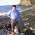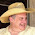## onsdag 9 juni 2010

### A Too Simplistic Climate Model

A simplistic climate model including effects of conduction, convection and radiation takes the form

R T + C T_x - RC T_xx = 0 for x in (0,1),  -  RC T_x(0) = Q, T(1) = small,

where T(x) is temperature at altitude x, T_x is the first and T_xx the second derivative with respect to x, R is a coefficient of net outgoing radiation, C is a convection velocity, RC is a
a combined conduction/radiation coefficient and Q (= 1 say) is a heat source.

Depending on the size of the coefficients R, C and RC, the temperature profile T(x) takes the following main forms:
• R = C = 0: T(x) = (1 - x)/RC :
• C = 0, A^2 = R/RC : T(x) = exp (-A x)
• RC = small: T(x) ~  exp(-R/C x).
We see a linearly decaying temperature profile with climate sensitivity T(0) = 1/RC,
or an exponentially decaying profile with T(0) = 1.

In the case of substantial net outgoing radiation with R not small and R/RC and R/C large, the exponential decay is rapid. If R/RC and R/C is small, then T(x) is almost constant.

The linear profile observed in reality is realized only in the first case with RC dominating R and C, and then with a large climate sensitivity if RC is small.

The above model is thus not easy to bend to fit with observation of a constant lapse rate which is not large, and thus seems too simplistic.

What the model is missing is the thermodynamics of an atmosphere under gravitation which
has an equilibrium (isentropic) state with constant not large lapse rate, as shown in posts on

We thus find that radiation/conduction must be combined with thermodynamics to describe the action of the atmopshere as a global air conditioner maintaining a surface temperature of 15 C under permanent heating from the Sun, including the crucial aspect of climate sensitivity.

The above argument gives yet another indication that a simplistic estimate of basic climate sensitivity of 1 C based on radiation only, may not be close to any reality.

#### 9 kommentarer:

1.加油！充實內函最重要！Beauty is but skin- deep...................................................

2.How did you derive this set of equations? They seem exceedingly odd. Normal climate models include the effect of gravitation through its effect on pressure (in fact pressure rather than altitude is often taken as the central variable for the 1-dimensional approximation).

3.This is a basic model including effects of convection, conduction and radiation, without gravitation et cet, intended to show that simplistic
models, like Stefan-Boltzmann, cannot be used to predict basic climate sensitivity.

4.Isn't that a straw man? Nobody is using SB to predict sensitivity directly.

The 1.2 C is simply the increase in temperature at the surface to balance 4 w/M^2 deficit, all else held exactly equal.

Of course, all else is not held equal, and this goes by the name "feedback" though I'm not convinced the analogy to control theory is all that strong.

Clearly the Clausius-Clapeyron condition acts to exacerbate the sensitivity. Other attenuating and exacerbating factors exist; according to existing simulation techniques they are dominated by clouds.

Most paleontological evidence points to, and almost all evidence is consistent with an amplification of about 2 to 3 fold, or a sensitivity around 3 C. The fact that simulations agree is not strong independent evidence, but does allow us to get some qualitative idea what the regional impacts would be under such a shift. This is not the primary scientific purpose of such simulations, but of course it is the most prominent one.

You appear to be determined to join Lindzen and Spencer in providing outlying estimates of the sensitivity that are small. To do so, constructing a full simulation will not suffice to be convincing, but it would be interesting to see such a thing.

If, however, you can provide a dynamical core that passes the usual dycore tests that is expressed in FEnICS, it would be an enormous service to earth and planetary sciences. We do need climate simulation models constructed according to contemporary software development techniques.

In general, Lindzen and Spencer (competitively) observationally estimate the sensitivity that are on spatial and time scales that are too short. Their approaches do not seem to be sound from what I understand, and they seem, as you do, very committed to getting a low number.

It would be great news if the number is actually low, but it doesn't seem likely. I don't understand the scientific basis for your or a few others' confidence in this matter.

5.See:

http://www-personal.umich.edu/~cjablon/dycore_test_suite.html

6.I have no predetermined idea of climate sensitivity, just try to understand.

Your statement that "The 1.2 C is simply the increase in temperature at the surface to balance 4 w/M^2 deficit, all else held exactly equal" is
precisly what I do not understand. From where is the 4 W, and from where comes the 1.2 C? What I understand is that 4 W of "radiative forcing" is an ad hoc assumption with unclear science behind, while 1.2 C is supposed to follow from SB. Right?

A first indication of small sensitivity is that the presence of the
entire atmosphere can be viewed to raise the Earth surface temp by 15 C (or 33 C depending what you consider to be an Earth without atmosphere).

The first guess of climate sensitivity would thus be 0.15 C from a 1% change of atmosphere (e.g. from doubled CO2). Right?

7.Claes, you claim this "model" includes the effects of radiation, but then (in the boundary condition/second equation) you only balance input energy Q with a "combined conduction/radiation coefficient", whatever that is supposed to mean - but in particular a term proportional only to the gradient of temperature, when in fact there is at least a component of outgoing radiative heat flux from the surface that depends only on temperature, and not on its gradient (the part that bypasses the atmosphere completely).

Furthermore, the surface energy balance and atmospheric energy balance terms are very different things due to the low absorptivity/emissivity of the atmosphere, but you seem to be lumping them together in your first equation. It makes no sense to me - but perhaps an explicit derivation would explain what you're talking about a bit better.

Michael Tobis - I get an error message going to the URL you provided, do you have a better one?

Finally, Claes Johnson, in your response to Michael Tobis, you claim:
"What I understand is that 4 W of "radiative forcing" is an ad hoc assumption with unclear science behind"

but this is completely false - see the Modtran software I pointed you to before for example. The physics of the 4 W/m^2 of radiative forcing is extremely sound.

And we've already talked about where the 1.2 C comes from (it's not "SB", it's from the specific radiative behavior of surface and atmosphere under a uniform temperature change; only the surface is "SB" to a good approximation).

And on your concluding argument why would doubled CO2 be a "1% change in the atmosphere"? Since CO2 is the essential driver of the greenhouse effect (see the Richard Alley talk I pointed you to earlier), doubling CO2 might naively suggest the 33 C basic greenhouse effect would itself be doubled. Luckily the effect is not linear in concentration of greenhouse gases.

If you are referring to the 4 W/m^2 radiative forcing being a "1% change", what is the 400 W/m^2 reference you are comparing to? There is no net heat flux of 400 W/m^2 anywhere in Earth's climate system. Total incoming solar energy reaching the surface is only 160 W/m^2, or 240 W/m^2 if you include surface and atmosphere, and that accounts for not just the 33 K Greenhouse effect, but the *entire* non-zero temperature of our planet! 4 W/m^2 is 1.7% of the 240 W/m^2 total incoming, so just multiplying 1.7% by the surface temperature of 288 K gives you 5 K of expected warming from doubling CO2 - if things were linear.

8.Re link, it was live when I posted it just yesterday. Here is Google's cache:

http://is.gd/cOTkG

9.Dear Arthur: I still consider "radiative forcing" to be unphysical since the heat energy absorbed by the Earth surface effectively comes from the Sun and not from any circular backradiation from the atmosphere: The heat radiated heat from the Earth surface only depends on the temperature drop to the medium absorbing the radiation (atmoshpere or empty space).

So if you claim that the physics behind 4 W/m2 of "radiative forccing" is "extremely sound" this is something I have to learn about and don't understand now. And I also have to learn about the 1.2 C "from the specific radiative behavior of surface and atmosphere" you speak of.
This material should be possible to find in any basic physics book or if not in IPCC AR4. Is it?